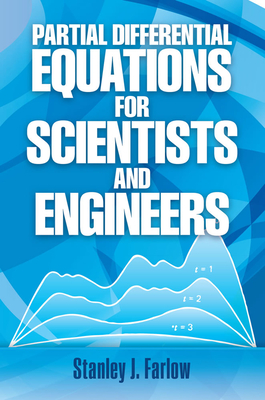Don't miss our 50% off Summer Sidewalk Gift Sale starting July 31 through August, while supplies last!

# Partial Differential Equations for Scientists and Engineers (Dover Books on Mathematics)# Partial Differential Equations for Scientists and Engineers (Dover Books on Mathematics)

\$ 18.95
Out of Stock In Store (Click to Order)

Most physical phenomena, whether in the domain of fluid dynamics, electricity, magnetism, mechanics, optics, or heat flow, can be described in general by partial differential equations. Indeed, such equations are crucial to mathematical physics. Although simplifications can be made that reduce these equations to ordinary differential equations, nevertheless the complete description of physical systems resides in the general area of partial differential equations.
This highly useful text shows the reader how to formulate a partial differential equation from the physical problem (constructing the mathematical model) and how to solve the equation (along with initial and boundary conditions). Written for advanced undergraduate and graduate students, as well as professionals working in the applied sciences, this clearly written book offers realistic, practical coverage of diffusion-type problems, hyperbolic-type problems, elliptic-type problems, and numerical and approximate methods. Each chapter contains a selection of relevant problems (answers are provided) and suggestions for further reading.

Partial Differential Equations & Beyond Stanley J. Farlow's Partial Differential Equations for Scientists and Engineers is one of the most widely used textbooks that Dover has ever published. Readers of the many Amazon reviews will easily find out why. Jerry, as Professor Farlow is known to the mathematical community, has written many other fine texts -- on calculus, finite mathematics, modeling, and other topics.We followed up the 1993 Dover edition of the partial differential equations title in 2006 with a new edition of his An Introduction toDifferential Equations and Their Applications. Readers who wonder if mathematicians have a sense of humor might search the internet for a copy of Jerry's The Girl Who Ate Equations for Breakfast (Aardvark Press, 1998). Critical Acclaim for Partial Differential Equations for Scientists and Engineers "This book is primarily intended for students in areas other than mathematics who are studying partial differential equations at the undergraduate level. The book is unusual in that the material is organized into 47 semi-independent lessonsrather than the more usual chapter-by-chapter approach. "An appealing feature of the book is the way in which the purpose of each lesson is clearly stated at the outset while the student will find the problems placed at the end of each lesson particularly helpful. The first appendix consists of integral transform tables whereas the second is in the form of a crossword puzzle which the diligent student should be able to complete after a thorough reading of the text. "Students (and teachers) in this area will find the book useful as the subject matter is clearly explained. The author and publishers are to be complimented for the quality of presentation of the material." -- K. Morgan, University College, Swansea

ISBN: 9780486676203

ISBN-10: 9780486676203

Publisher: Dover Publications

Publication Date: 09/01/1993 - 12:00am

On Sale: 09/01/1993 - 12:00am

Pages: 448

Language: English